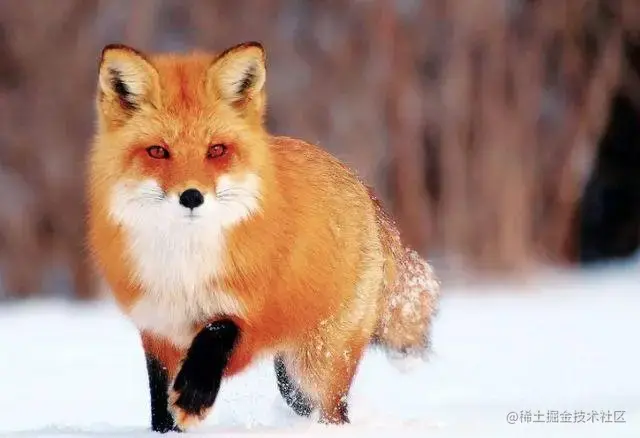# Keras深度學習——使用對抗攻擊生成可欺騙神經網路的影象

## 2. 使用 Keras 實現對抗攻擊

img = cv2.imread('5.png') img = cv2.cvtColor(img, cv2.COLOR_BGR2RGB) plt.imshow(img) plt.show() ``` 影象的視覺化結果如下所示：# 將影象畫素值歸一化至[-1,1]

original_image = img.astype('float')/255 original_image -= 0.5 original_image *= 2.

# 維度擴充套件

original_image = np.expand_dims(original_image, axis=0) `匯入預訓練的模型，並使用此模型預測影象中物件的類別：`python from keras.preprocessing import image from keras.applications import inception_v3 model = inception_v3.InceptionV3() predictions = model.predict(original_image) predicted_classes = inception_v3.decode_predictions(predictions, top=1) imagnet_id, name, confidence = predicted_classes print("This is a {} with {:.4}% confidence".format(name, confidence * 100)) `模型對於輸入的影象，輸出的預測結果如下，看以看到模型可以以很高的置信度得到正確的結果：`python This is a red_fox with 92.09% confidence `接下來，為對抗攻擊模型定義輸入和輸出：`python model_input_layer = model.layers.input model_output_layer = model.layers[-1].output ````model_input_layer` 是模型的輸入，而 `model_output_layer` (具有 `softmax` 啟用函式的最後一層)是輸入影象屬於各種類別的概率。 設定原始影象的修改限制(即圖片最大變化閾值)，指定了修改原始影象應遵照的限制：```python max_change_above = np.copy(original_image) + 0.01 max_change_below = np.copy(original_image) - 0.01 adversarial_sample = np.copy(original_image) `初始化損失函式，以便模型將修改後影象預測為山貓(模型輸出預測向量中的第 `283` 個索引值)：`python learning_rate = 0.1 prob_cat = []

# 山貓

object_type_to_fake = 283 cost_function = model_output_layer[0, object_type_to_fake] ````model_output_layer` 的輸出是影象的各種類別的概率。在本例中，我們指定損失函式由我們要偽造物件類別的索引位置決定。 初始化損失相對於輸入的梯度函式：```python gradient_function = K.gradients(cost_function, model_input_layer) ````` 此程式碼計算```cost_function`相對於`model_input_layer` (輸入影象)的梯度變化。

while cost < 0.80: cost, gradients = grab_cost_and_gradients_from_model([adversarial_sample, 0]) adversarial_sample += gradients * learning_rate adversarial_sample = np.clip(adversarial_sample, max_change_below, max_change_above) prob_cat.append(cost) print("Model's predicted likelihood that the image is an tiger cat: {:.8}%".format(cost * 100)) `在以上程式碼中，我們獲得了與輸入影象 (`adversarial_sample`) 相對應的損失和梯度資訊。另外，我們利用梯度(乘以學習率)更新輸入影象。最後，如果被修改的圖片超過了預定義的圖片最大變化閾值，我們會對其進行裁剪，以確保影象修改前後的變化並不明顯。不斷迴圈執行這些步驟，直到獲得輸入影象至少有 `0.8` 的概率被預測為山貓為止。`shell Model's predicted likelihood that the image is an tiger cat: 0.0043381504% Model's predicted likelihood that the image is an tiger cat: 0.0043390162% ... Model's predicted likelihood that the image is an tiger cat: 74.044788% Model's predicted likelihood that the image is an tiger cat: 89.34136% ```